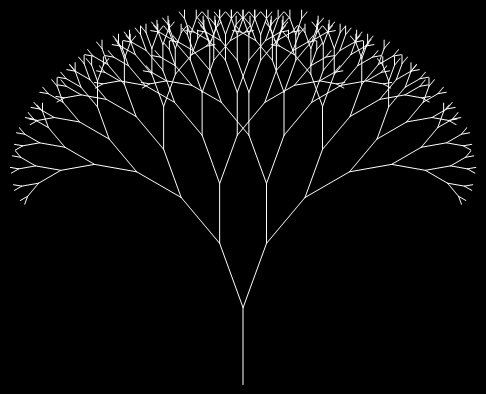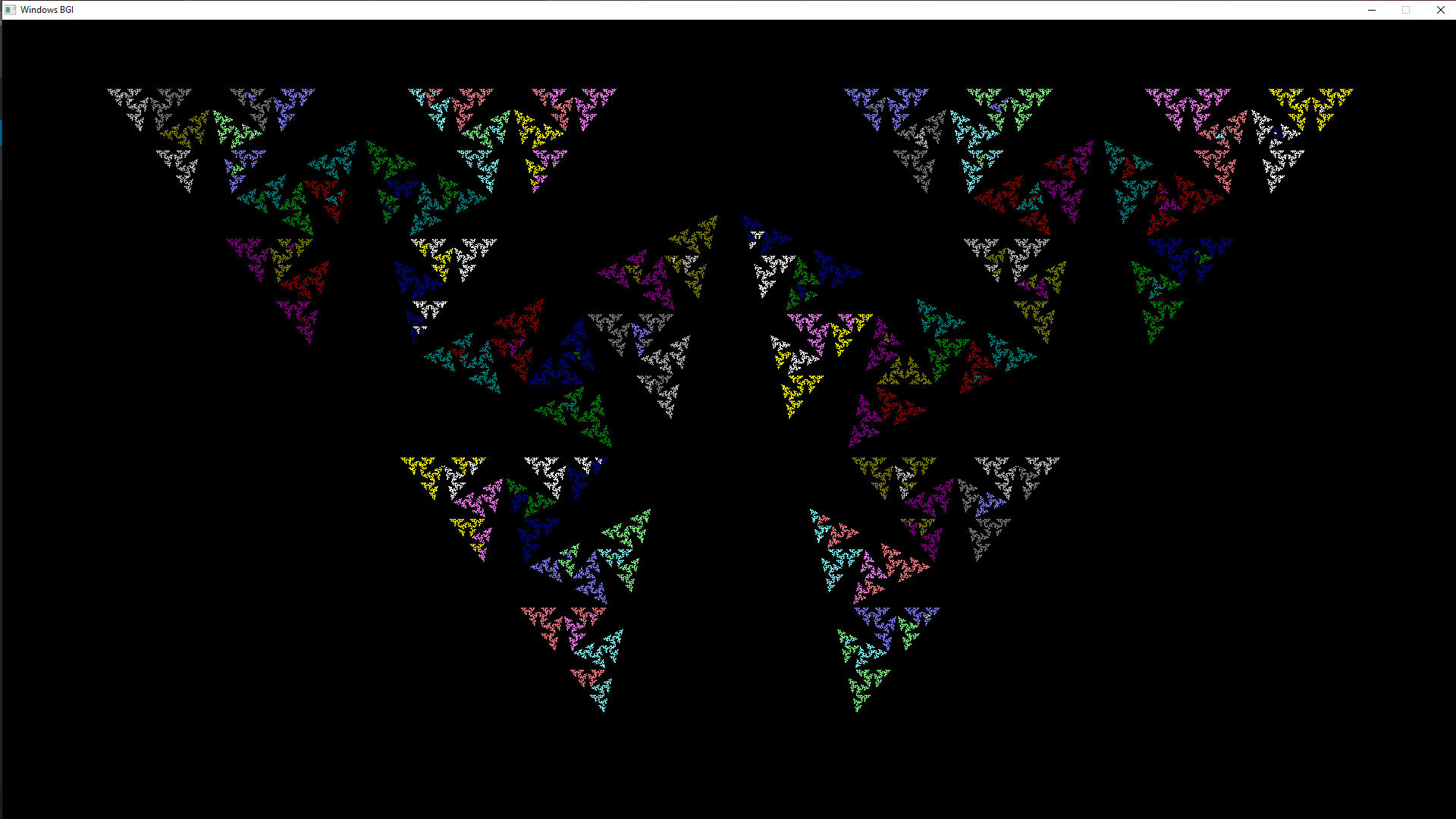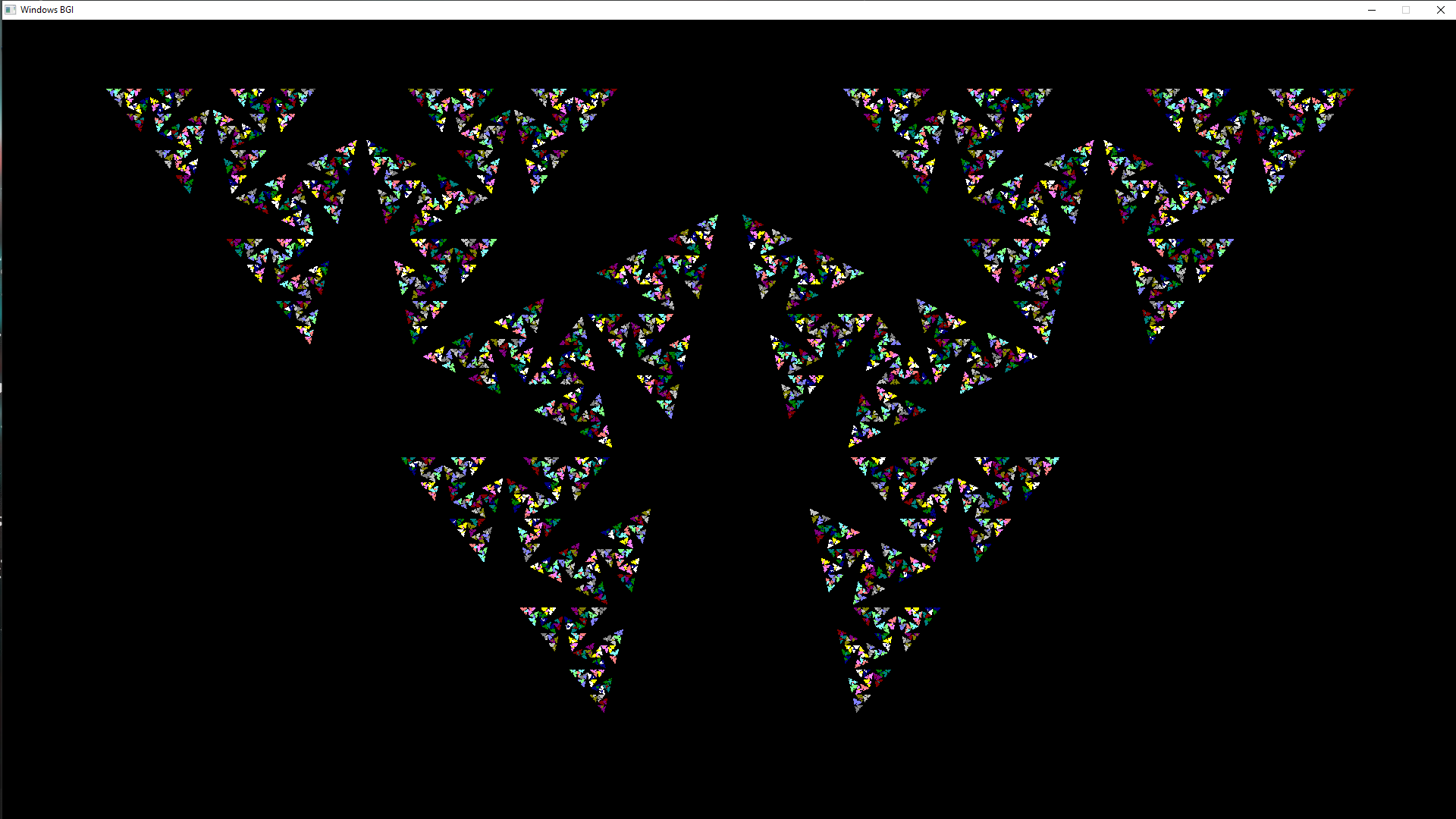# Creating Butterfly themed Fractal in C++ Using Graphics

• Last Updated : 16 Dec, 2019

In Layman’s terms, Fractals are beautiful patterns brought into existence upon the intertwining of computation and mathematics. To get a bit technical they are recursive in nature such that when looked upon a certain subset of a given fractal a similar pattern appears to emerge out of it.In this article, a new type of fractal pattern is generated, given as below:Approach:

1. Recursively go to the end branch of a Fractal tree.
2. When the length of the branch reaches our threshold value, draw out that particular branch and exit out of the function.
3. To keep the colors similar for a few iterations, we use type casting since ((colorVal%15000)/1000+1) would give out spectrums of same values, i.e. same colour thousand times before it iterates.

Below is the implementation of the above approach:

 `// C++ code to Create a Butterfly ``// themed Fractal using Graphics`` ` `#include ``#include ``#include `` ` `#define Y 1080``#define X 1920`` ` `// c is defined as the angle between each``// iterative lines, changing this would result``// in different and interesting patterns.``#define c 5 * M_PI / 7`` ` `// colorVal is used to provide different colors``// for each iterating point/line in the cycle.``int` `colorVal = 0;`` ` `// The core function in the program which is``// recursive in nature and terminates when the``// line size is less than 0.1 pixels.``int` `Pyt_Tree(``float` `x, ``float` `y, ``float` `r, ``float` `ang)``{`` ` `    ``// Max iteration condition so as to increase``    ``// the accuracy of the fractal pattern.``    ``// The closer it is to zero the higher the``    ``// details but would cost more processing time.``    ``if` `(r < 0.1) {`` ` `        ``// combination of type casting and iteration``        ``// so as to provide a Greatest Integer Function``        ``// sort of manipulation for the color input.``        ``setcolor((colorVal++ % 15000) / 1000 + 1);`` ` `        ``// Conversion of the parametric coordinates``        ``// of the points to the Argand coordinates``        ``// while displaying them.``        ``line(x, y, x - r * ``sin``(ang), y - r * ``cos``(ang));`` ` `        ``return` `0;``    ``}`` ` `    ``float` `r_n;`` ` `    ``if` `(c > M_PI / 4) {``        ``r_n = ``sin``(c);``    ``}``    ``else` `{``        ``r_n = ``cos``(c);``    ``}`` ` `    ``// Recursive calling of the Pyt_Tree() function``    ``// to get towards the end of the branch of the``    ``// fractal tree.``    ``Pyt_Tree(x - r * ``sin``(ang), y - r * ``cos``(ang),``             ``r / (2 * r_n), (ang + c));``    ``Pyt_Tree(x - r * ``sin``(ang), y - r * ``cos``(ang),``             ``r / (2 * r_n), (ang - c));`` ` `    ``return` `0;``}`` ` `// Driver code``int` `main()``{``    ``initwindow(X, Y);``    ``Pyt_Tree(X / 2, Y, Y * 0.9, 0);``    ``getch();``    ``closegraph();``    ``return` `0;``}`

Output:My Personal Notes arrow_drop_up